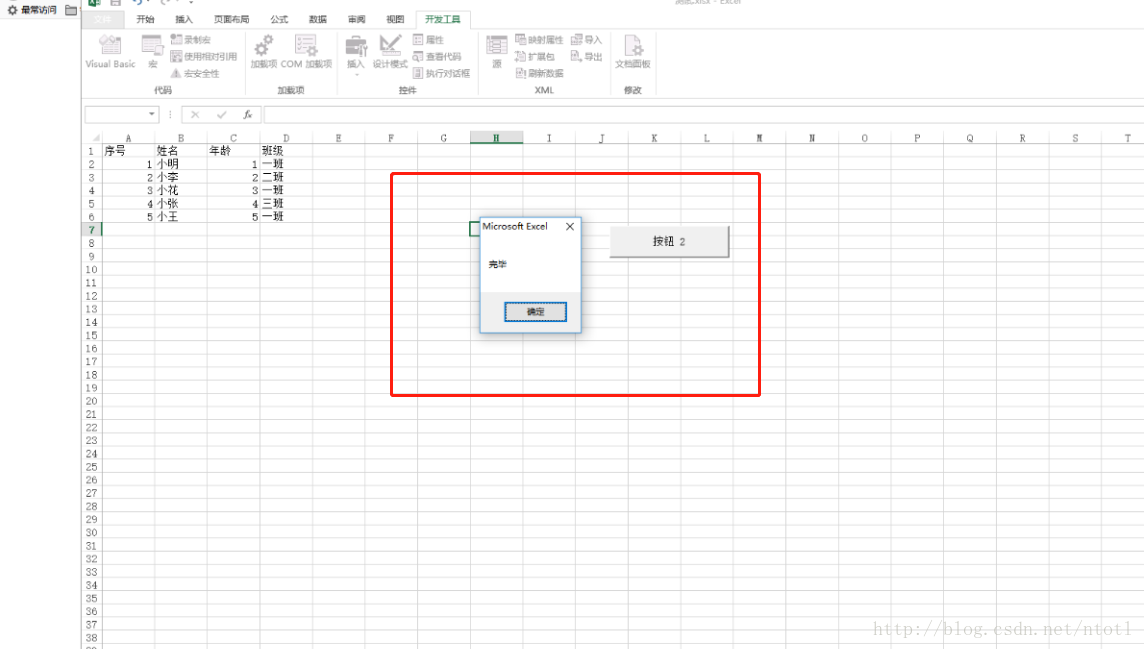﻿ vba将excel按照某一列拆分成多个文件_vbs_脚本之家

# vba将excel按照某一列拆分成多个文件

更新时间：2023年01月29日 10:34:43   作者：ntotl

1.打开目标excel,按alt + f11键打开VBE窗口2.选择插入->模块粘贴下面代码到编辑器中Sub 保留表头拆分数据为若干新工作簿()

```    Dim arr, d As Object, k, t, i&, lc%, rng As Range, c%
c = Application.InputBox("请输入拆分列号", , 4, , , , , 1)
If c = 0 Then Exit Sub
Application.ScreenUpdating = False
arr = [a1].CurrentRegion
lc = UBound(arr, 2)
Set rng = [a1].Resize(, lc)
Set d = CreateObject("scripting.dictionary")
For i = 2 To UBound(arr)
If Not d.Exists(arr(i, c)) Then
Set d(arr(i, c)) = Cells(i, 1).Resize(1, lc)
Else
Set d(arr(i, c)) = Union(d(arr(i, c)), Cells(i, 1).Resize(1, lc))
End If
Next
k = d.Keys
t = d.Items
For i = 0 To d.Count - 1
rng.Copy .Sheets(1).[a1]
t(i).Copy .Sheets(1).[a2]
.SaveAs Filename:=ThisWorkbook.Path & "\" & k(i) & ".xls"
.Close
End With
Next
Application.ScreenUpdating = True
MsgBox "完毕"
End Sub```3.保存后回到excel文件，选择开发工具->插入->表单控件(按钮(窗体控件))，没有开发工具选项的在设置里面打开选项如图4.按住鼠标左键不动然后在excel中划定一个按钮区域，然后会自动弹出的窗口，选择刚才保存的宏，点击确定5.鼠标从按钮上面移开  ，然后点击按钮输入要列号，就是根据哪列的数据进行拆分文件，下图我们输入4就是按照班级进行拆分，根据不同的班级拆分成不同的文件6.点击确定后，如果弹出完毕提示框代表拆分完成7.到源文件所在的目录文件中查看拆分的文件